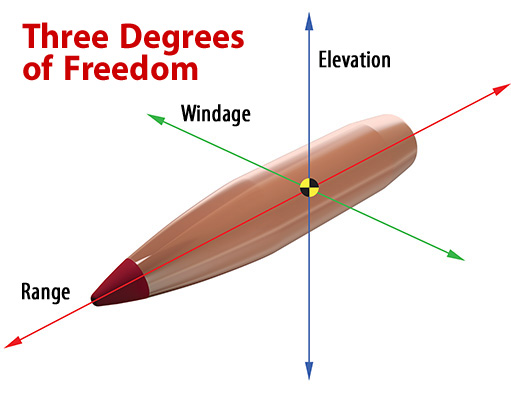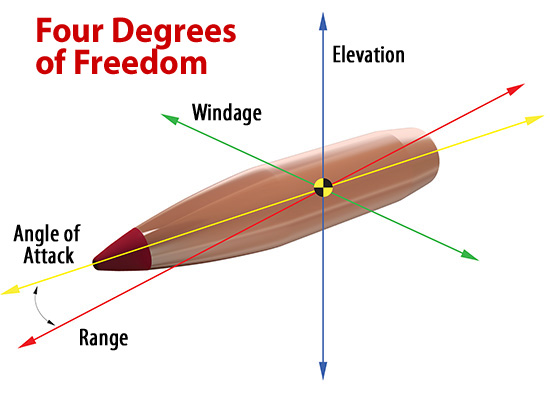# Overview

The Hornady® 4DOF® (Four Degrees Of Freedom) Ballistic Calculator determines trajectory solutions based on projectile Drag Coefficient (not Ballistic Coefficient), combined with exact physical modeling of the projectile and its mass and aerodynamic properties. Additionally, it is the first publicly available calculator that will accurately determine the correct vertical shift a bullet experiences as it encounters a crosswind; referred to as Aerodynamic Jump (AJ).

The Hornady 4DOF® also accurately calculates angled shots by accounting for important conditions that current programs overlook. The use of Drag Coefficient and the calculation of Aerodynamic Jump enable the Hornady 4DOF® Ballistic Calculator to be the most accurate trajectory calculator commercially available.

## The 4DOF® & Degrees Of Freedom

Quite simply, "Degree or Degrees Of Freedom” is a physics term for actions or responses of an object that are independent of each other. Up to this point, all publicly offered small arms trajectory programs have treated a projectile as an inanimate lump with only three degrees of freedom used to calculate trajectory (windage, elevation, and range); the projectile’s movement in relation to its line of flight isn’t accounted for.The trajectory solution provided by the Hornady 4DOF® Ballistic Calculator is calculated by incorporating the projectile’s movement in the standard 3 degrees as discussed above, as well as its movement about its center of gravity and subsequent angle relative to its line of flight (angle of attack), aka the 4th Degree Of Freedom. A tremendous amount of the software, as well as projectile properties, goes into the calculation of this simple sounding angle of attack.## Moving Away from the Ballistic Coefficient

Why calculate a trajectory using a mathematical comparison of your bullet to a “Standard Projectile” (oversimplification of BC) when you can use an exact model of your projectile in the trajectory calculation (Drag Coefficient)?  Using Doppler radar, Hornady ballisticians have calculated the exact drag versus velocity curve for each projectile in the 4DOF® Bullet Library. Ballistic Coefficient can change as velocity changes. A drag curve doesn’t change; the curve is specific to each projectile and is directly related to its trajectory.

The Hornady 4DOF® Ballistic Calculator accurately calculates the mass properties, aerodynamic properties and the responses of the projectile as it flies downrange as well as the movements of the projectile due to these responses such as spin drift, accurate Gyroscopic Stability (Sg) and Aerodynamic Jump (AJ). There is no Ballistic Coefficient (BC) needed or used in 4DOF®.